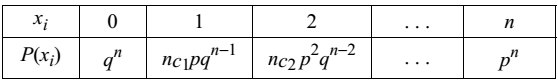# Binomial Distribution

This was discovered by a Swiss Mathematician James Bernoulli (1654-1705).

### Bernoulli’s Trials

Consider a random experiment that has only two possible outcomes. For example when a coin is tossed you can take the falling of head as success and falling of tail as failure. Assume that these outcomes have probabilities p and q respectively such that p + q = 1.

If the experiment is repeated n times independently with two possible outcomes, they are called Bernoulli’s trials. A Binomial distribution can be used under the following condition.

• (i) Any trial, result in a success or a failure
• (ii) There are a finite number of trials which are independent
• (iii) The probability of success is the same in each trial

### Probability Function

Let n be a given positive integer and p be a given real number such that 0 ≤ p ≤ 1. Also let q = 1 − p.The table is called the Binomial distribution.

Binomial probability function B(n,p,x) gives the probability of exactly x successes in n Bernoullian trials, p being the probability of success in a trial. The constants n and p are called the parameters of the distribution.

### Constants of Binomial Distribution

Mean = np

Variance = npq

Standard deviation = √(variance) = √(npq)

In a Binomial distribution mean is always greater than the variance.